Question

1) The power factor correction impedance is placed in parallel with the load. Can it be installed in series? Justify your answer.

2) What happens to the power supplied by the source before
and after power factor correction? Why, in the absence of power factor correction, does the source need to deliver more power to the circuit to maintain the voltage at the load? Justify your answer in terms of reactive power, and line losses.

We need at least 10 more requests to produce the answer.

0 / 10 have requested this problem solution

The more requests, the faster the answer.

All students who have requested the answer will be notified once they are available.

#### Earn Coins

Coins can be redeemed for fabulous gifts.

Similar Homework Help Questions
• ### Question: A three-phase line has an impedance of 1 + j2 Ω per phase. The line...

Question: A three-phase line has an impedance of 1 + j2 Ω per phase. The line feeds two balanced three-phase loads that are connected in parallel. The first load is Y-connected and has an impedance of 20+j40 Ω per phase. The second load is ∆-connected and has an impedance of 30-j60 Ω per phase. The line-to-line voltage at the load end of the line is 415V. Taking the phase voltage Va as reference, determine: a) The total current per phase...

• ### 1. (50 Points) angle phase voltage source supplies an RL load connected in parallel configuration throu...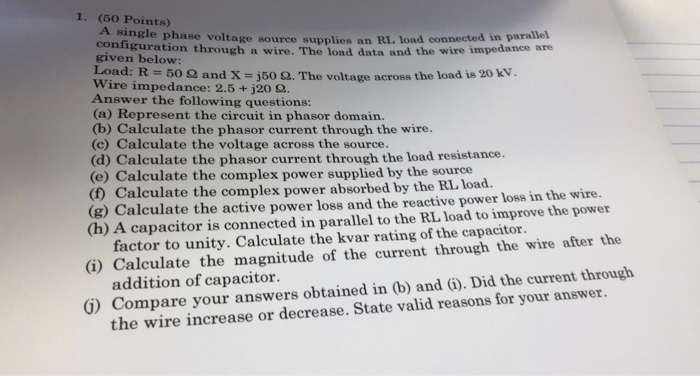1. (50 Points) angle phase voltage source supplies an RL load connected in parallel configuration throu given below: Load: R 500 and X j50 Ω. The voltage across the load is 20 kV. wire impedance : 2.5 +j20 Ω. Answer the following questions: (a) Represent the circuit in phasor domain. (b) Calculate the phasor current through the wire. (c) Calculate the voltage across the source. (d) Calculate the phasor current through the load resistance. (e) Calculate the complex power supplied...

• ### 1) Figure- generator is producing a line voltage of 480 V, and the line impedance is...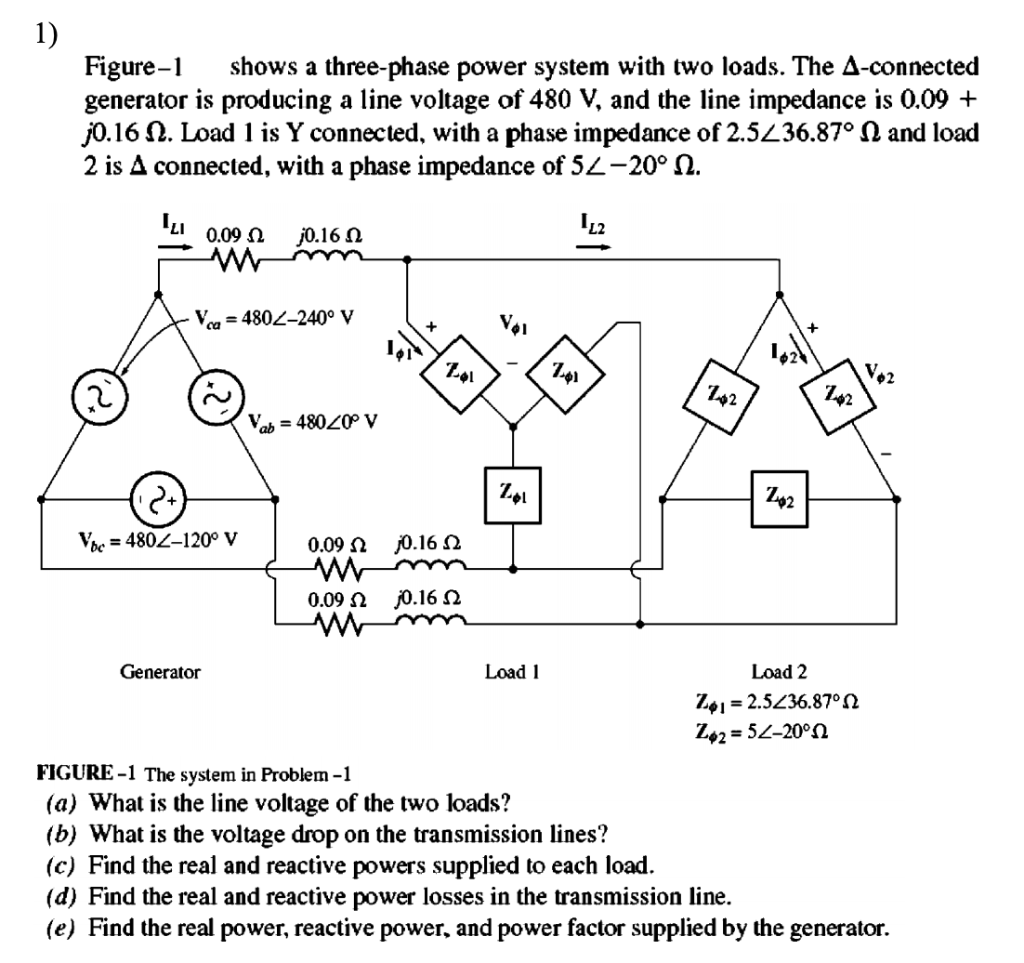1) Figure- generator is producing a line voltage of 480 V, and the line impedance is 0.09 + j0.16 . Load 1 is Y connected, with a phase impedance of 2.5236.87° 0 and load 2 is A connected, with a phase impedance of 54-20° n. shows a three-phase power system with two loads. The A-connected 2 0.09 j0.16 n w Vca= 4802-240° V + Vab = 48020 V Vhc = 480-120° V 0.09 j0.16 0.09 j0.16 Load 1 Load 2...

• ### Balanced wye-connected load with a per-phase impedance....

A balanced wye-connected load with a per-phase impedance of (4+j3)ohms is supplied by a 173-V, 60Hz three-phase sourcea. Find the line current, the power factor, and the total volt-amperes, real power, and reactive power absorbed by the load.Please provide your work so I can follow the steps taken to answer each. Thanks Life Saver!

• ### UISIZULU Problem 4 A three phase, 5 kW, wye-connected load operate onnected load operates at 0.8...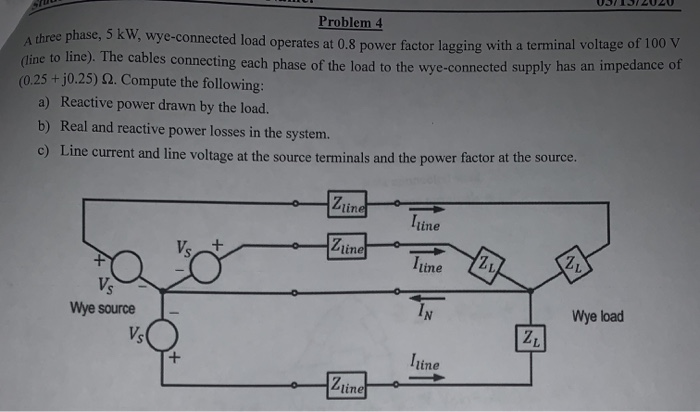UISIZULU Problem 4 A three phase, 5 kW, wye-connected load operate onnected load operates at 0.8 power factor lagging with a terminal voltage of 100 V (line to line). The cables connecting e bles connecting each phase of the load to the wye-connected supply has an impedance of (0.25 +0.25) 12. Compute the following: a) Reactive power drawn by the load. b) Real and reactive power losses in the system. me current and line voltage at the source terminals and...

• ### 1. A load (Z-10+j5) is supplied using a transmission line with an impedance of (Z-1+2j). Calculate...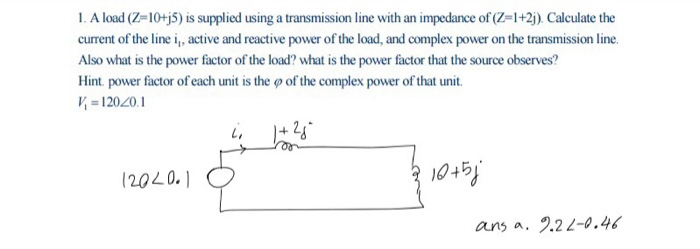1. A load (Z-10+j5) is supplied using a transmission line with an impedance of (Z-1+2j). Calculate the curent of the line i, active and reactive power of the load, and complex power on the transmission line Also what is the power factor of the load? what is the power factor that the source observes? Hint. power factor of each unit is the p of the complex power of that unit 120.20.1 10451 (202 0.1 ans a. 2.22-0.46

• ### 4. In the circuit below, the impedance Z1 dissipates 10 kW with a power factor of...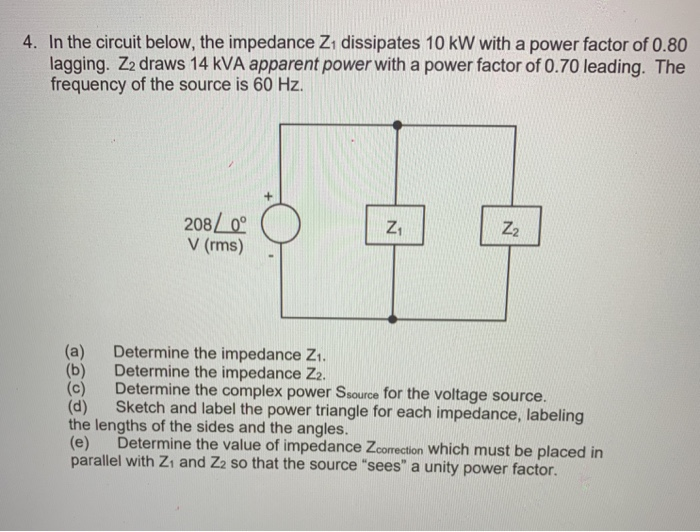4. In the circuit below, the impedance Z1 dissipates 10 kW with a power factor of 0.80 lagging. Z2 draws 14 kVA apparent power with a power factor of 0.70 leading. The frequency of the source is 60 Hz 208 V (rms)- Z1 (a) Determine the impedance Zi. (b) Determine the impedance Z2. (c) Determine the complex power Ssource for the voltage source. (d) Sketch and label the power triangle for each impedance, labeling the lengths of the sides and...

• ### A three-phase line with an impedance of (0.25+j1.25) ohms/phase supplies power to three balanced 3-phase parallel...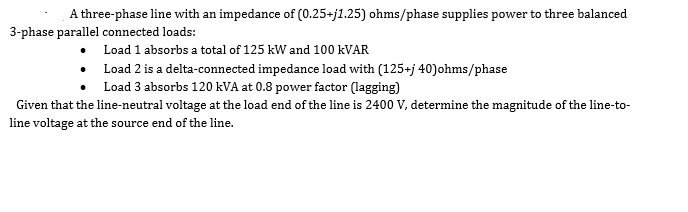A three-phase line with an impedance of (0.25+j1.25) ohms/phase supplies power to three balanced 3-phase parallel connected loads: • Load 1 absorbs a total of 125 kW and 100 KVAR • Load 2 is a delta-connected impedance load with (125+j 40)ohms/phase • Load 3 absorbs 120 kVA at 0.8 power factor (lagging) Given that the line-neutral voltage at the load end of the line is 2400 V, determine the magnitude of the line-to- line voltage at the source end of...

• ### -An electric load consists of a 4Ω resistance, 6Ω inductive reactance and 9Ω capacitive reactance connected...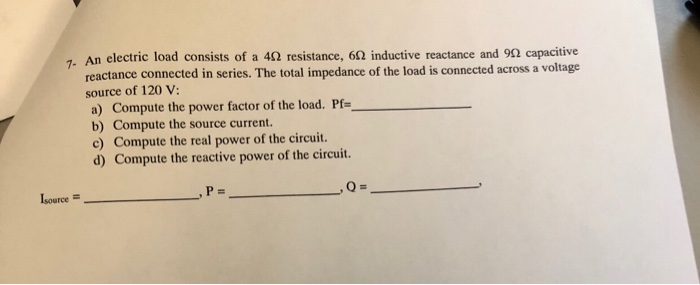-An electric load consists of a 4Ω resistance, 6Ω inductive reactance and 9Ω capacitive reactance connected in series. The total impedance of the load is connected across a voltage source of 120 V: a) Compute the power factor of the load. Pf- b) Compute the source current c) Compute the real power of the circuit. d) Compute the reactive power of the circuit. Isource

• ### Power Calculations 4 of 4 Review Learning Goal In this trial, you will practice the callation...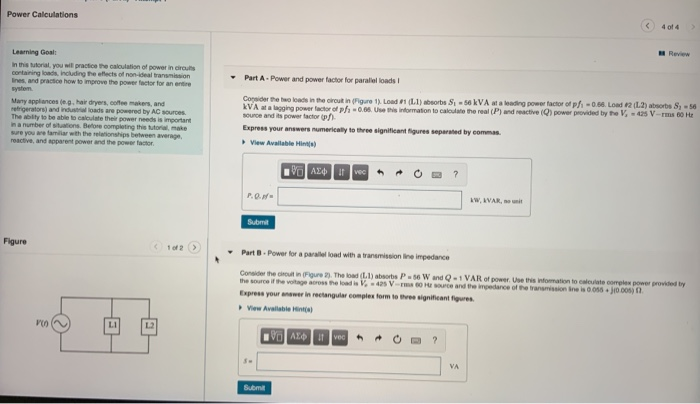Power Calculations 4 of 4 Review Learning Goal In this trial, you will practice the callation of power in circuts cortaring loads, including the effects of non-ideal transmission Ines, and practice how to improve the power factor for an entire system. Many appliancese.. hair dryers, coffee makers, and refrigerators) and indoads are powered by AC sources The folly to be able to calculate the power needs is important ma number of towns. Before completing the Moral make sure you are...